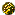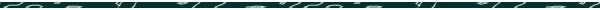Seven Reasons Why the Prophetic Solar Year
is Set to the Day of Y2K

(IN DETAIL)

Why Y2K?Summarized   In detail:  (The following documents are only in draft form.)IntroductionReasons One to ThreeReason Number Four (a & b)Reason Number Five (a)Reason Number Five (b)Reason Number Six & SevenConclusion and Endnotes

DefinitionsThe bible says that a prophetic year is 360 days long.As deduced from the bible, a prophetic solar year is both 365.242 189 670 139 and 365.242 189 669 536 days long---the average being 365.242 189 669 8 days. (Note that these figures are virtually identicalendar)A "broadening cycle" is a cycle that is larger than its base cycle. For instance, the 4000-year cycle is a broader cycle than its smaller 40-year cycle, and the 96000-year cycle is broader than it's 4000 cycle, etc.Click here for, What does an "average" solar year mean?When we speak of "millions of years," this does not mean that the earth itself has lasted, or will last, millions of years, but only that the prophetic clock gives the appearance of being eternal. The prophetic clock reveals the God of all ages, but not the age of God's Creation. The present heavens and earth may pass away within as little as 1000 years (Rev. 20; 21:1).

Introduction

As deduced from the bible, a prophetic solar year is both 365.242 189 670 139 and 365.242 189 669 536 days long---the average being 365.242 189 669 8 days (or, 365.242 189 669 837 5 to be exact.)

The first figure produces broadening cycles of (1 year times) 40 x 100 x 24 x 150 x 24 (= 345,600,000-year cycles). This completes one cycle so that "A thousand years is like one day." On this scale, 345,600,000 years symbolically equals 960 years.  The exact day that marks the solar year of 365.242189670139 necessary to produce this "1000-year Calendar" occurred just two days BEFORE Y2K. (This is explained as we continue; Y2K was Jan. 1st, 2000.)

The second figure of 365.242189669536 produces a calendar that simply continues the 1000-year calendar, except on into eternity. The exact day that marks the solar year of 365.242189669536 necessary to produce the Eternal Calendar occurred just two days AFTER Y2K. This is why the two solar-year figures are essentially the same.

Y2K (Jan. 1st, 2000) was therefore the middle or average of the above two days, with the solar year at 365.2421896698 days. (This can be verified by simply doing a search for 365.2421896698 in a search engine on the Net, and noting the Julian day number assigned to it.)

This is the prophetic calendar of which we are examining, and the question before us is: How is it that only the day of Y2K has the exact average orbiting speed of the earth around the sun whereby the resulting solar year (if made constant) would produce all of the numerous bible-number patterns?

See, "Reasons One to Three," to continue.Why Y2K?Summarized   In detail: (The following documents are only in draft form.)IntroductionReasons One to ThreeReason Number Four (a & b)Reason Number Five (a)Reason Number Five (b)Reason Number Six & SevenConclusion and Endnotes

New-Years Day on the 360 Calendar